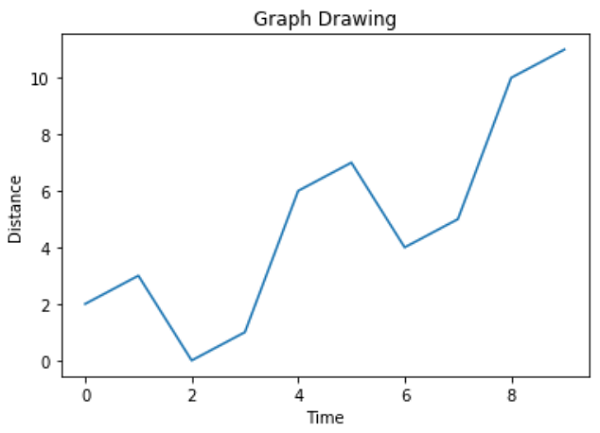# Python - Measuring Central Tendency

Mathematically central tendency means measuring the center or distribution of location of values of a data set. It gives an idea of the average value of the data in the data set and also an indication of how widely the values are spread in the data set. That in turn helps in evaluating the chances of a new input fitting into the existing data set and hence probability of success.

There are three main measures of central tendency which can be calculated using the methods in pandas python library.

• Mean - It is the Average value of the data which is a division of sum of the values with the number of values.

• Median - It is the middle value in distribution when the values are arranged in ascending or descending order.

• Mode - It is the most commonly occurring value in a distribution.

## Calculating Mean and Median

The pandas functions can be directly used to calculate these values.

```import pandas as pd

#Create a Dictionary of series
d = {'Name':pd.Series(['Tom','James','Ricky','Vin','Steve','Smith','Jack',
'Lee','Chanchal','Gasper','Naviya','Andres']),
'Age':pd.Series([25,26,25,23,30,29,23,34,40,30,51,46]),
'Rating':pd.Series([4.23,3.24,3.98,2.56,3.20,4.6,3.8,3.78,2.98,4.80,4.10,3.65])}

#Create a DataFrame
df = pd.DataFrame(d)
print "Mean Values in the Distribution"
print df.mean()
print "*******************************"
print "Median Values in the Distribution"
print df.median()
```

Its output is as follows −

```Mean Values in the Distribution
Age       31.833333
Rating     3.743333
dtype: float64
*******************************
Median Values in the Distribution
Age       29.50
Rating     3.79
dtype: float64
```

## Calculating Mode

Mode may or may not be available in a distribution depending on whether the data is continous or whether there are values which has maximum frquency. We take a simple distribution below to find out the mode. Here we have a value which has maximum frequency in the distribution.

```import pandas as pd

#Create a Dictionary of series
d = {'Name':pd.Series(['Tom','James','Ricky','Vin','Steve','Smith','Jack',
'Lee','Chanchal','Gasper','Naviya','Andres']),
'Age':pd.Series([25,26,25,23,30,25,23,34,40,30,25,46])}
#Create a DataFrame
df = pd.DataFrame(d)

print df.mode()
```

Its output is as follows −```     Age      Name
0   25.0    Andres
1    NaN  Chanchal
2    NaN    Gasper
3    NaN      Jack
4    NaN     James
5    NaN       Lee
6    NaN    Naviya
7    NaN     Ricky
8    NaN     Smith
9    NaN     Steve
10   NaN       Tom
11   NaN       Vin
```

## Useful Video Courses

Video

#### Python Online Training

187 Lectures 17.5 hours

Video

#### Python Essentials Online Training

55 Lectures 8 hours

Video

#### Learn Python Programming in 100 Easy Steps

136 Lectures 11 hours

Video

#### Python with Data Science

Best Seller

75 Lectures 13 hours

Video

#### Python 3 from scratch to become a developer in demand

Best Seller

70 Lectures 8.5 hours

Video

#### Python Data Science basics with Numpy, Pandas and Matplotlib

Most Popular

63 Lectures 6 hours